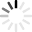# Area Quizzes & Trivia

A squared plus B squared equals … no, that’s not right. The square root of B-square minus four times ... no, that’s not it either. Area is a measure of the size of a two-dimensional surface that a particular shape takes up, and there’s no single equation that will get you the right answer for any particular shapes!

From triangles to trapezoids, there’s a trick to finding the area of any given polygon – and half the battle is knowing which formula to use in the first place! If you need practice telling the difference between a rhombus and a rectangle, or just need a little practice with your calculator to get the right answer each time, give these area quizzes a try!
Top Trending

Use the information given to find the area of the circle.  Use the following formula: Area = radius squared times pi. (pi = 3.14)

Questions: 10  |  Attempts: 6189   |  Last updated: Jan 22, 2013
• Sample Question
The radius of a circle is 3 inches. What is the area?Finding the area of triangles, parallelograms trapezoids etc.

Questions: 15  |  Attempts: 1757   |  Last updated: Nov 11, 2020
• Sample Question
What do you call the measurement around the outside of a figure?Use the formulas from today's notes to complete the following examples.

Questions: 5  |  Attempts: 4760   |  Last updated: May 11, 2021
• Sample Question
Find the area of the following.Take the assigned math quiz.  This will be a good practice for your quiz in class.

Questions: 6  |  Attempts: 3674   |  Last updated: Jan 9, 2013
• Sample Question
1.  What does the letter b stand for in the formula  A = bhMotor and Central Systems p. 130-133

Questions: 14  |  Attempts: 920   |  Last updated: Jan 24, 2013
• Sample Question
Select the incorrect match.Related